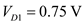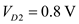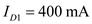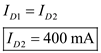Problem

# Two diodes are in series. The first diode has a voltage of 0.75 V and the second has a vol...

Two diodes are in series. The first diode has a voltage of 0.75 V and the second has a voltage of 0.8 V. If the current through the first diode is 400 mA, what is the current through the second diode?

#### Step-by-Step Solution

Solution 1

It is given that,

The two diodes are in series.

The first diode voltage isThe second diode voltage isThe current through first diode isIn series circuit the current passing through elements is same. That is, if two components are series the current passing through those two components are same.

The two diodes are connected in series. Thus,

The current passing through second diode is,Add your Solution
Textbook Solutions and Answers Search
Solutions For Problems in Chapter 3